## 1 Introduction

Inspired by service-oriented computing, Microservices structure software applications as highly modular and scalable compositions of fine-grained and loosely-coupled services . These features support modern software engineering practices, like continuous delivery/deployment  and application autoscaling . Currently, these practices focus on single microservices and do not take advantage of the information on the interdependencies within an architecture. On the contrary, architecture-level deployment supports the global optimization of resource usage and avoids “domino” effects due to unstructured scaling actions that may cause cascading slowdowns or outages [27, 35, 39].

In this paper, we formalize the problem of automatic deployment and reconfiguration (at the architectural level) of microservice systems, proving formal properties and presenting an implemented solution.

In our work, we follow the approach taken by the Aeolus component model [13,14,15], which was used to formally define the problem of deploying component-based software systems and to prove that, in the general case, such problem is undecidable . The basic idea of Aeolus is to enrich the specification of components with a finite state automaton that describes their deployment life cycle. Previous work identified decidable fragments of the Aeolus model: e.g., removing from Aeolus replication constraints (e.g., used to specify a minimal amount of services connected to a load balancer) makes the deployment problem decidable, but non-primitive recursive ; removing also conflicts (e.g., used to express the impossibility to deploy in the same system two types of components) makes the problem PSpace-complete  or even poly-time , but under the assumption that every required component can be (re)deployed from scratch.

Our intuition is that the Aeolus model can be adapted to formally reason on the deployment of microservices. To achieve our goal, we significantly revisit the formalization of the deployment problem, replacing Aeolus components with a model of microservices. The main difference between our model of microservices and Aeolus components lies in the specification of their deployment life cycle. Here, instead of using the full power of finite state automata (like in Aeolus and other TOSCA-compliant deployment models ), we assume microservices to have two states: (i) creation and (ii) binding/unbinding. Concerning creation, we use strong dependencies to express which microservices must be immediately connected to newly created ones. After creation, we use weak dependencies to indicate additional microservices that can be bound/unbound. The principle that guided this modification comes from state-of-the-art microservice deployment technologies like Docker  and Kubernetes . In particular, the weak and strong dependencies have been inspired by Docker Compose  (a language for defining multi-container Docker applications) where it is possible to specify different relationships among microservices using, e.g., the depends_on (resp. external_links) modalities that force (resp. do not force) a specific startup order similarly to our strong (resp. weak) dependencies. Weak dependencies are also useful to model horizontal scaling, e.g., a load balancer that is bound to/unbound from many microservice instances during its life cycle.

In addition, w.r.t. the Aeolus model, we also consider resource/cost-aware deployments, taking inspiration from the memory and CPU resources found in Kubernetes. Microservice specifications are enriched with the amount of resources they need to run. In a deployment, a system of microservices runs within a set of computation nodes. Nodes represent computational units (e.g., virtual machines in an Infrastructure-as-a-Service Cloud deployment). Each node has a cost and a set of resources available to the microservices it hosts.

On the model above, we define the optimal deployment problem as follows: given an initial microservice system, a set of available nodes, and a new target microservice to be deployed, find a sequence of reconfiguration actions that, once applied to the initial system, leads to a new deployment that includes the target microservice. Such a deployment is expected to be optimal, meaning that the total cost (i.e., the sum of the costs) of the nodes used is minimal. We show that this problem is decidable by presenting an algorithm working in three phases: (1) generate a set of constraints whose solution indicates the microservices to be deployed and their distribution over the nodes; (2) generate another set of constraints whose solution indicates the connections to be established; (3) synthesize the corresponding deployment plan. The set of constraints includes optimization metrics that minimize the overall cost of the computed deployment.

The algorithm has NEXPTIME complexity because, in the worst-case, the length of the deployment plan could be exponential in the size of the input. However, we consider this worst-case unfeasible in practice, as the number of microservices deployable on one node is limited by the available resources. Under the assumption that each node can host at most a polynomial amount of microservices, the deployment problem is NP-complete and the problem of deploying a system minimizing its total cost is an NP-optimization problem. Moreover, having reduced the deployment problem in terms of constraints, we can exploit state-of-the-art constraint solvers [12, 23, 24] that are frequently used in practice to cope with NP-hard problems.

To concretely evaluate our approach, we consider a real-world microservice architecture, inspired by the reference email processing pipeline from Iron.io . We model that architecture in the Abstract Behavioral Specification (ABS) language, a high-level object-oriented language that supports deployment modeling . We use our technique to compute two types of deployments: an initial one, with one instance for each microservice, and a set of deployments to horizontally scale the system depending on small, medium or large increments in the number of emails to be processed. The experimental results are encouraging in that we were able to compute deployment plans that add more than 30 new microservice instances, assuming availability of hundreds of machines of three different types, and guaranteeing optimality.

## 2 The Microservice Optimal Deployment Problem

We model microservice systems as aggregations of components with ports. Each port exposes provided and required interfaces. Interfaces describe offered and required functionalities. Microservices are connected by means of bindings indicating which port provides the functionality required by another port. As discussed in the Introduction, we consider two kinds of requirements: strong required interfaces, that need to be already fulfilled when the microservice is created, and weak required interfaces, that must be fulfilled at the end of a deployment (or reconfiguration) plan. Microservices are enriched with the specification of the resources they need to properly run; such resources are provided to the microservices by nodes. Nodes can be seen as the unit of computation executing the tasks associated to each microservice.

As an example, in Fig. 1 we have reported the representation of the deployment of a microservice system inspired by the email processing pipeline that we will discuss in Sect. 3. Here, we consider a simplified pipeline. A Message Receiver microservice handles inbound requests, passing them to a Message Analyzer that checks the email content and sends the attachments for inspection to an Attachment Analyzer. The Message Receiver has a port with a weak required interface that can be fulfilled by Message Analyzer instances. This requirement is weak, meaning that the Message Receiver can be initially deployed without any connection to instances of Message Analyzer. These connections can be established afterwards and reflect the possibility to horizontally scale the application by adding/removing instances of Message Analyzer. This last microservice has instead a port with a strong required interface that can be fulfilled by Attachment Analyzer instances. This requirement is strong to reflect the need to immediately connect a Message Analyzer to its Attachment Analyzer.

Figure 1 presents a reconfiguration that, starting from the initial deployment depicted in continuous lines, adds the elements depicted with dashed lines. Namely, a couple of new instances of Message Analyzer and a new instance of Attachment Analyzer are deployed. This is done in order to satisfy numerical constraints associated to both required and provided interfaces. For required interfaces, the numerical constraints indicate lower bounds to the outgoing bindings, while for provided interfaces they specify upper bounds to the incoming connections. Notice that the constraint $$\ge 3$$ associated to the weak required interface of Message Receiver is not initially satisfied; this is not problematic because constraints on weak interfaces are relevant only at the end of a reconfiguration. In the final deployment, such a constraint is satisfied thanks to the two new instances of Message Analyzer. These two instances need to be immediately connected to an Attachment Analyzer: only one of them can use the initially available Attachment Analyzer, because of the constraint $$\le 2$$ associated to the corresponding provided interface. Hence, a new instance of Attachment Analyzer is added.

We also model resources: each microservice has associated resources that it consumes (see the CPU and RAM quantities associated to the microservices in Fig. 1). Resources are provided by nodes, that we represent as containers for the microservice instances, providing them the resources they require. Notice that nodes have also costs: the total cost of a deployment is the sum of the costs of the used nodes (e.g., in the example the total cost is 598 cents per hour, corresponding to the cost of 4 nodes: 2 C4 large and 2 C4 xlarge virtual machine instances of the Amazon public Cloud).

We now move to the formal definitions. We assume the following disjoint sets: $$\mathcal {I}$$ for interfaces, $$\mathcal {Z}$$ for microservices, and a finite set $$\mathcal {R}$$ for kinds of resources. We use $${\mathbb {N}}$$ to denote natural numbers, $${\mathbb {N}}^+$$ for $${\mathbb N}\setminus \{0\}$$, and $${\mathbb {N}}^+_{\infty }$$ for $${\mathbb {N}}^+\cup \{\infty \}$$.

### Definition 1

(Microservice type). The set $${\varGamma }$$ of microservice types, ranged over by $$\mathcal {T}_1,\mathcal {T}_2,\ldots$$, contains 5-ples $$\langle P, D_s, D_w, C, R\rangle$$ where:

•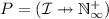are the provided interfaces, defined as a partial function from interfaces to corresponding numerical constraints (indicating the maximum number of connected microservices);

•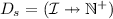are the strong required interfaces, defined as a partial function from interfaces to corresponding numerical constraints (indicating the minimum number of connected microservices);

•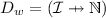are the weak required interfaces (defined as the strong ones, with the difference that also the constraint 0 can be used indicating that it is not strictly necessary to connect microservices);

• $$C \subseteq \mathcal {I}{}$$ are the conflicting interfaces;

• $$R = (\mathcal {R}{} \rightarrow {\mathbb {N}})$$ specifies resource consumption, defined as a total function from resources to corresponding quantities indicating the amount of required resources.

We assume sets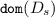,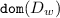and C to be pairwise disjoint.Footnote 1

Notation: given a microservice type $$\mathcal {T} = \langle P, D_s, D_w, C, R\rangle$$, we use the following postfix projections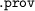,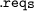,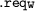,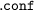andto decompose it; e.g.,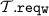returns the partial function associating arities to weak required interfaces. In our example, for instance, the Message Receiver microservice type is such that Message Receiver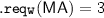and Message Receiver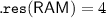. When the numerical constraints are not explicitly indicated, we assume as default value $$\infty$$ for provided interfaces (i.e., they can satisfy an unlimited amount of ports requiring the same interface) and 1 for required interfaces (i.e., one connection with a port providing the same interface is sufficient).

Inspired by , we allow a microservice to specify a conflicting interface that, intuitively, forbids the deployment of other microservices providing the same interface. Conflicting interfaces can be used to express conflicts among microservices, preventing both of them to be present at the same time, or cases in which only one microservice instance can be deployed (e.g., a consistent and available microservice that can not be replicated).

Since the requirements associated with strong interfaces must be immediately satisfied, it is possible to deploy a configuration with circular dependencies only if at least one weak required interface is involved in the cycle. In fact, having a cycle with only strong required interfaces would mean to deploy all the microservices involved in the cycle simultaneously. We now formalize a well-formedness condition on microservice types to guarantee the absence of such configurations.

### Definition 2

(Well-formed Universe). Given a finite set of microservice types U (that we also call universe), the strong dependency graph of U is as follows: $$G(U)=(U,V)$$ with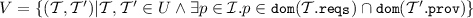. The universe U is well-formed if G(U) is acyclic.

In the following, we always assume universes to be well-formed. Well-formedness does not prevent the specification of microservice systems with circular dependencies, which are captured by cycles with at least one weak required interface.

### Definition 3

(Nodes). The set $$\mathcal {N}$$ of nodes is ranged over by $$o_1,o_2,\ldots$$ We assume the following information to be associated to each node o in $$\mathcal {N}$$.

• A function $$R = (\mathcal {R}{} \rightarrow {\mathbb {N}})$$ that specifies node resource availability: we use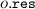to denote such a function.

• A value in $${\mathbb {N}}$$ that specifies node cost: we use $$o$$ to denote such a value.

As example, in Fig. 1, the node Node1_large is such that Node1_large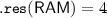and Node1_large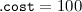.

We now define configurations that describe systems composed of microservice instances and bindings that interconnect them. A configuration, ranged over by $${\mathcal {C}}_1, {\mathcal {C}}_2,\ldots$$, is given by a set of microservice types, a set of deployed microservices (with their associated type), and a set of bindings. Formally:

### Definition 4

(Configuration). A configuration $$\mathcal {C}$$ is a 4-ple $$\langle Z, T, N, B\rangle$$ where:

• $$Z \subseteq \mathcal {Z}$$ is the set of the currently deployed microservices;

• $$T = (Z \rightarrow \mathcal {T})$$ are the microservice types, defined as a function from deployed microservices to microservice types;

• $$N = (Z \rightarrow \mathcal {N})$$ are the microservice nodes, defined as a function from deployed microservices to nodes that host them;

• $$B \subseteq \mathcal {I}\times Z \times Z$$ is the set of bindings, namely 3-ples composed of an interface, the microservice that requires that interface, and the microservice that provides it; we assume that, for $$(p,z_1,z_2) \in B$$, the two microservices $$z_1$$ and $$z_2$$ are distinct and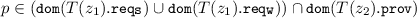.

In our example, if we use mr to refer to the instance of Message Receiver, and ma for the initially available Message Analyzer, we will have the binding (MA, mr, ma). Moreover, concerning the microservice placement function N, we have $$N(\mathsf{mr})= \mathsf{Node1\_large}$$ and $$N(\mathsf{ma})= \mathsf{Node2\_xlarge}$$.

We are now ready to formalize the notion of correctness of configuration. We first define a provisional correctness, considering only constraints on strong required and provided interfaces, and then we define a general notion of configuration correctness, considering also weak required interfaces and conflicts. The former is intended for transient configurations traversed during the execution of a reconfiguration, while the latter for the final configuration.

### Definition 5

(Provisionally correct configuration). A configuration $${\mathcal {C}} \!=\! \langle Z, T, N, B\rangle$$ is provisionally correct if, for each node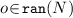, it holdsFootnote 2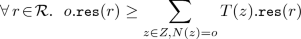and, for each microservice $$z \in Z$$, both following conditions hold:

•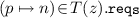implies that there exist n distinct microservices $$z_1,\ldots , z_n \in \!Z \!\setminus \! \{z\}$$ such that, for every $$1 \le i \le n$$, we have $$\langle p, z, z_i\rangle \in B$$;

•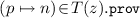implies that there exist no m distinct microservices $$z_1,\ldots , z_m \in Z \!\setminus \! \{z\}$$, with $$m > n$$, such that, for every $$1 \le i \le m$$, we have $$\langle p, z_i, z\rangle \in B$$.

### Definition 6

(Correct configuration). A configuration $${\mathcal {C}} \!=\! \langle Z, T, N, B\rangle$$ is correct if $${\mathcal {C}}$$ is provisionally correct and, for each microservice $$z \in Z$$, both following conditions hold:

•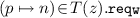implies that there exist n distinct microservices $$z_1,\ldots , z_n \in \!Z \!\setminus \! \{z\}$$ such that, for every $$1 \le i \le n$$, we have $$\langle p, z, z_i\rangle \in B$$;

•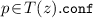implies that, for each $$z' \in Z\!\setminus \!\{z\}$$, we have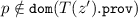.

Notice that, in the example in Fig. 1, the initial configuration (in continuous lines) is only provisionally correct in that the weak required interface MA (with arity 3) of the Message Receiver is not satisfied (because there is only one outgoing binding). The full configuration — including also the elements in dotted lines — is instead correct: all the constraints associated to the interfaces are satisfied.

We now formalize how configurations evolve by means of atomic actions.

### Definition 7

(Actions). The set $$\mathcal {A}$$ contains the following actions:

• $$bind (p,z_1,z_2)$$ where $$z_1,z_2 \!\in \! \mathcal {Z}$$, with $$z_1 \!\ne \! z_2$$, and $$p \!\in \! \mathcal {I}$$: add a binding between $$z_1$$ and $$z_2$$ on port p (which is supposed to be a weak-require port of $$z_1$$ and a provide port of $$z_2$$);

• $$unbind (p,z_1,z_2)$$ where $$z_1,z_2 \!\in \! \mathcal {Z}$$, with $$z_1 \!\ne \! z_2$$, and $$p \!\in \! \mathcal {I}$$: remove the specified binding on p (which is supposed to be a weak required interface of $$z_1$$ and a provide port of $$z_2$$);

• $$new (z,{\mathcal {T}}, o, B_s)$$ where $$z \!\in \! \mathcal {Z}$$, $${\mathcal {T}} \!\in \! \Gamma$$, $$o \!\in \! \mathcal {N}$$ and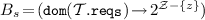; with $$B_s$$ (representing bindings from strong required interfaces in $$\mathcal {T}$$ to sets of microservices) being such that, for each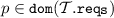, it holds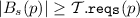: add a new microservice z of type $$\mathcal {T}$$ hosted in o and bind each of its strong required interfaces to a set of microservices as described by $$B_s$$;Footnote 3

• $$del (z)$$ where $$z \!\in \! \mathcal {Z}$$: remove the microservice z from the configuration and all bindings involving it.

In our example, assuming that the initially available Attachment Analyzer is named aa, we have that the action to create the initial instance of Message Analyzer is $$new (\mathsf{ma},\mathsf{Message Analyzer}, \mathsf{Node2\_xlarge}, (\mathsf{AA} \mapsto \{\mathsf{aa}\}))$$. Notice that it is necessary to establish the binding with the Attachment Analyzer because of the corresponding strong required interface.

The execution of actions can now be formalized using a labeled transition system on configurations, which uses actions as labels.

### Definition 8

(Reconfigurations). Reconfigurations are denoted by transitions $$\mathcal {C} \xrightarrow {\alpha } \mathcal {C}'$$ meaning that the execution of $$\alpha \in \mathcal {A}$$ on the configuration $$\mathcal {C}$$ produces a new configuration $$\mathcal {C}'$$. The transitions from a configuration $$\mathcal {C}=\langle Z,T,N,B \rangle$$ are defined as follows:

A deployment plan is simply a sequence of actions that transform a provisionally correct configuration (without violating provisional correctness along the way) and, finally, reach a correct configuration.

### Definition 9

(Deployment plan). A deployment plan $$\mathsf {P}$$ from a provisionally correct configuration $${\mathcal C}_{0}$$ is a sequence of actions $$\alpha _{1}, \dots , \alpha _{m}$$ such that:

• there exist $${\mathcal C}_{1}, \dots , {\mathcal C}_{m}$$ provisionally correct configurations, with $${\mathcal C}_{i-1} \xrightarrow {\alpha _{i}} {\mathcal C}_{i}$$ for $$1 \le i \le m$$, and

• $${\mathcal C}_{m}$$ is a correct configuration.

Deployment plans are also denoted with $${\mathcal C}_{0} \xrightarrow {\alpha _1} {\mathcal C}_{1} \xrightarrow {\alpha _{2}} \cdots \xrightarrow {\alpha _{m}} {\mathcal C}_{m}$$.

In our example, a deployment plan that reconfigures the initial provisionally correct configuration into the final correct one is as follows: a $$new$$ action to create the new instance of Attachment Analyzer, followed by two $$new$$ actions for the new Message Analyzers (as commented above, the connection with the Attachment Analyzer is part of these $$new$$ actions), and finally two $$bind$$ actions to connect the Message Receiver to the two new instances of Message Analyzer.

We now have all the ingredients to define the optimal deployment problem, that is our main concern: given a universe of microservice types, a set of available nodes and an initial configuration, we want to know whether and how it is possible to deploy at least one microservice of a given microservice type $$\mathcal {T}$$ by optimizing the overall cost of nodes hosting the deployed microservices.

### Definition 10

(Optimal deployment problem). The optimal deployment problem has, as input, a finite well-formed universe U of microservice types, a finite set of available nodes O, an initial provisionally correct configuration $${\mathcal C}_{0}$$ and a microservice type $$\mathcal {T}_t \in U$$. The output is:

• A deployment plan $$\mathsf {P} = {\mathcal C}_{0} \xrightarrow {\alpha _1} {\mathcal C}_{1} \xrightarrow {\alpha _{2}} \cdots \xrightarrow {\alpha _{m}} {\mathcal C}_{m}$$ such that

• for all $$\mathcal {C}_{i}=\langle Z_i,T_i,N_i,B_i \rangle$$, with $$1 \le i \le m$$, it holds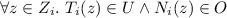, and

• $$\mathcal {C}_{m}=\langle Z_m,T_m,N_m,B_m \rangle$$ satisfies $$\exists z \in Z_m : T_i(z) = \mathcal {T}_t$$;

if there exists one. In particular, among all deployment plans satisfying the constraints above, one that minimizes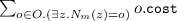(i.e., the overall cost of nodes in the last configuration $${\mathcal C}_{m}$$), is outputted.

• no (stating that no such plan exists); otherwise.

We are finally ready to state our main result on the decidability of the optimal deployment problem. To prove the result we describe an approach that splits the problem in three incremental phases: (1) the first phase checks if there is a possible solution and assigns microservices to deployment nodes, (2) the intermediate phase computes how the microservices need to be connected to each other, and (3) the final phase synthesizes the corresponding deployment plan.

### Theorem 1

The optimal deployment problem is decidable.

### Proof

The proof is in the form of an algorithm that solves the optimal deployment problem. We assume that the input to the problem to be solved is given by U (the microservice types), O (the set of available nodes), $${\mathcal C}_{0}$$ (the initial provisionally correct configuration), and $$\mathcal {T}_t \in U$$ (the target microservice type). We use $$\mathcal {I}(U)$$ to denote the set of interfaces used in the considered microservice types, namely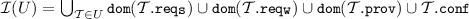.

The algorithm is based on three phases.

Phase 1 The first phase consists of the generation of a set of constraints that, once solved, indicates how many instances should be created for each microservice type $$\mathcal {T}$$ (denoted with $$\texttt {inst}(\mathcal {T})$$), how many of them should be deployed on node o (denoted with $$\texttt {inst}(\mathcal {T},o)$$), and how many bindings should be established for each interface p from instances of type $$\mathcal {T}$$ — considering both weak and strong required interfaces — and instances of type $$\mathcal {T}'$$ (denoted with $$\texttt {bind}(p,\mathcal {T},\mathcal {T}')$$). We also generate an optimization function that guarantees that the generated configuration is minimal w.r.t. its total cost.

We now incrementally report the generated constraints. The first group of constraints deals with the number of bindings:

Constraint 1a and 1b guarantee that there are enough bindings to satisfy all the required interfaces, considering both strong and weak requirements. Symmetrically, constraint 1c guarantees that the number of bindings is not greater than the total available capacity, computed as the sum of the single capacities of each provided interface. In case the capacity is unbounded (i.e., $$\infty$$), it is sufficient to have at least one instance that activates such port to support any possible requirement (see constraint 1d). Finally, constraint 1e guarantees that no binding is established connected to provided interfaces of microservice types that are not deployed.

The second group of constraints deals with the number of instances of microservices to be deployed.

The first constraint 2a guarantees the presence of at least one instance of the target microservice. Constraint 2b guarantees that no two instances of different types will be created if one activates a conflict on an interface provided by the other one. Constraint 2c, consider the other case in which a type activates the same interface both in conflicting and provided modality: in this case, at most one instance of such type can be created. Finally, the constraints 2d and 2e guarantee that there are enough pairs of distinct instances to establish all the necessary bindings. Two distinct constraints are used: the first one deals with bindings between microservices of two different types, the second one with bindings between microservices of the same type.

The last group of constraints deals with the distribution of microservice instances over the available nodes O.

Constraint 3a simply formalizes the relationship among the variables $$\texttt {inst}(\mathcal {T})$$ and $$\texttt {inst}(\mathcal {T},o)$$ (the total amount of all instances of a microservice type, should correspond to the sum of the instances locally deployed on each node). Constraint 3b checks that each node has enough resources to satisfy the requirements of all the hosted microservices. The last two constraints define the optimization function used to minimize the total cost: constraint 3c introduces the boolean variable $$\texttt {used}(o)$$ which is true if and only if node o contains at least one microservice instance; constraint 3d is the function to be minimized, i.e., the sum of the costs of the used nodes.

These constraints, and the optimization function, are expected to be given in input to a constraint/optimization solver. If a solution is not found it is not possible to deploy the required microservice system; otherwise, the next phases of the algorithm are executed to synthesize the optimal deployment plan.

Phase 2 The second phase consists of the generation of another set of constraints that, once solved, indicates the bindings to be established between any pair of microservices to be deployed. More precisely, for each type $$\mathcal {T}$$ such that $$\texttt {inst}(\mathcal {T})>0$$, we use $$s_i^\mathcal {T}$$, with $$1 \le i \le \texttt {inst}(\mathcal {T})$$, to identify the microservices of type $$\mathcal {T}$$ to be deployed. We also assume a function N that associates microservices to available nodes O, which is compliant with the values $$\texttt {inst}(\mathcal {T},o)$$ already computed in Phase 1, i.e., given a type $$\mathcal {T}$$ and a node o, the number of $$s_i^\mathcal {T}$$, with $$1 \le i \le \texttt {inst}(\mathcal {T})$$, such that $$N(s_i^\mathcal {T}) = o$$ coincides with $$\texttt {inst}(\mathcal {T},o)$$.

In the constraints below we use the variables $$\texttt {b}(p,s_i^\mathcal {T},s_j^{\mathcal {T}'})$$ (with $$i \ne j$$, if $$\mathcal {T}={\mathcal {T}'}$$): its value is 1 if there is a connection between the required interface p of $$s_i^\mathcal {T}$$ and the provided interface p of $$s_j^{\mathcal {T}'}$$, 0 otherwise. We use n and m to denote $$\texttt {inst}(\mathcal {T})$$ and $$\texttt {inst}(\mathcal {T}')$$, respectively, and an auxiliary total function $${limProv}(\mathcal {T}',p)$$ that extends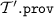associating 0 to interfaces outside its domain.

Constraint 4a considers the provided interface capacities to fix upper bounds to the bindings to be established, while constraints 4b and 4c fix lower bounds based on the required interface capacities, considering both the weak (see 4b) and the strong (see 4c) ones. Finally, constraint 4d indicates that it is not possible to establish connections on interfaces that are not required.

A solution for these constraints exists because, as also shown in , the constraints 1a $$\dots$$ 2e (already solved during Phase 1) guarantee that the configuration to be synthesized contains enough capacity on the provided interfaces to satisfy all the required interfaces.

Phase 3 In this last phase we synthesize the deployment plan that, when applied to the initial configuration $${\mathcal C}_{0}$$, reaches a new configuration $${\mathcal C}_{t}$$ with nodes, microservices and bindings as computed in the first two phases of the algorithm. Without loss of generality, in this decidability proof we show the existence of a simple plan that first removes the elements in the initial configuration and then deploys the target configuration from scratch. However, as also discussed in Sect. 3, in practice it is possible to define more complex planning mechanisms that re-use microservices already deployed.

Reaching an empty configuration is a trivial task since it is always possible to perform in the initial configuration unbind actions for all the bindings connected to weak required interfaces. Then, the microservices can be safely deleted. Thanks to the well-formedness assumption (Definition 2) and using a topological sort, it is possible to order the microservices to be removed without violating any strong required interface (e.g., first remove the microservice not requiring anything and repeat until all the microservices have been deleted).

The deployment of the target configuration follows a similar pattern. Given the distribution of microservices over nodes (computed in the first phase) and the corresponding bindings (computed in the second phase), the microservices can be created by following a topological sort considering the microservices dependencies following from the strong required interfaces. When all the microservices are deployed on the corresponding nodes, the remaining bindings (on weak required ports) may be added in any possible order.   $$\square$$

### Remark 1

The constraints generated during Phase 2 of the algorithm, in order to establish the microservice bindings, are expected to be given in input to a constraint/optimization solver. One can enrich such constraints with metrics to optimize, e.g., the number of local bindings (i.e., give a preference to the connections among microservices hosted in the same node):

$$\min \sum _{\mathcal {T},\mathcal {T}' \in U, i \in 1 \ldots \texttt {inst}(\mathcal {T}), j \in 1 \ldots \texttt {inst}(\mathcal {T}'), p \in \mathcal {I}(U), N(s_i^\mathcal {T}) \ne N(s_j^{\mathcal {T}'})} \texttt {b}(p,{s_i^\mathcal {T}},s_j^{\mathcal {T}'})$$

Another example, used in the case study discussed in Sect. 3, is the following metric that maximizes the number of bindingsFootnote 4:

$$\max \sum _{s_i^\mathcal {T}, s_j^{\mathcal {T}'}, p \in \mathcal {I}(U)} \texttt {b}(p,s_i^\mathcal {T}, s_j^{\mathcal {T}'})$$

From the complexity point of view, it is possible to show that the decision versions of the optimization problem solved in Phase 1 is NP-complete, in Phase 2 is in NP, while the planning in Phase 3 is synthesized in polynomial time. Unfortunately, due to the fact that numeric constraints can be represented in log space, the output of Phase 2 requiring the enumeration of all the microservices to deploy can be exponential in the size of the output of Phase 1 (indicating only the total number of instances for each type). For this reason, the optimal deployment problem is in NEXPTIME. However, we consider unfeasible in practice the deployment of an exponential number of microservices on one node having limited resources. If at most a polynomial number of microservices can be deployed on each node, we have that the optimal deployment problem becomes an NP-optimization problem and its decision version is NP-complete. See the companion technical report  for the formal proofs of complexity.

## 3 Application of the Technique to the Case-Study

Given the asymptotic complexity of our solution (NP under the assumption of polynomial size of the target configuration) we have decided to evaluate its applicability in practice by considering a real-world microservice architecture, namely the email processing pipeline described in . The considered architecture separates and routes the components found in an email (headers, links, text, attachments) into distinct, parallel sub-pipelines with specific tasks (e.g., remove malicious attachments, tag the content of the mail). We report in Fig. 2 a depiction of the architecture. When an email reaches the Message Receiver it is forwarded to the Message Parser, which sends each component into a specific sub-pipeline. In the sub-pipelines, some microservices — e.g., Text Analyzer and Attachment Analyzer — coordinate with other microservices — e.g., Sentiment Analyzer and Virus Scanner — to process their inputs. Each microservice in the architecture has a given resource consumption (expressed in terms of CPU and memory). As expected, the processing of each email component entails a specific load. Some microservices can handle large inputs, e.g., in the range of 40K simultaneous requests (e.g., Header Analyzer that processes short and uniform inputs). Other microservices sustain heavier computations (e.g., Image Recognizer) and can handle smaller simultaneous inputs, e.g., in the range of 10K requests.

To model the system above, we use the Abstract Behavioral Specification (ABS) language, a high-level object-oriented language that supports deployment modeling . ABS is agnostic w.r.t. deployment platforms (Amazon AWS, Microsoft Azure) and technologies (e.g., Docker or Kubernetes) and it offers high-level deployment primitives for the creation of new deployment components and the instantiation of objects inside them. Here, we use ABS deployment components as computation nodes, ABS objects as microservice instances, and ABS object references as bindings. Finally, to describe the requirements in our model, we use ABS with SmartDepl , an extension that supports deployment annotations. Strong required interfaces are modeled as class annotations indicating mandatory parameters for the class constructor: such parameters contain the references to the objects corresponding to the microservices providing the strongly required interfaces. Weak required interfaces are expressed as annotations concerning specific methods used to pass, to an already instantiated object, the references to the objects providing the weakly required interfaces. We define a class for each microservice type, plus one load balancer class for each microservice type. A load balancer distributes requests over a set of instances that can scale horizontally. Finally, we model nodes corresponding to Amazon EC2 instances: c4_large, c4_xlarge, and c4_2xlarge (with the corresponding provided resources and costs).

Initial (10K)

+20K

+50K

+80K

MessageReceiver ( $$\infty$$ )

1

-

-

-

MessageParser (40K)

1

-

+1

-

1

-

+1

-

1

-

+1

-

TextAnalyzer (15K)

1

+1

+2

+2

SentimentAnalyzer (15K)

1

+3

+4

+6

AttachmentsManager (30K)

1

+1

+2

+2

VirusScanner (13K)

1

+3

+4

+6

ImageAnalyzer (30K)

1

+1

+2

+2

NSFWDetector (13K)

1

+3

+4

+6

ImageRecognizer (13K)

1

+3

+4

+6

MessageAnalyzer (70K)

1

+1

+2

+2

In the table above, we report the result of our algorithm w.r.t. four incremental deployments: the initial in column 2 and under incremental loads in 3–5. We also consider an availability of 40 nodes for each of the three node types. In the first column of the Table, next to a microservice type, we report its corresponding maximum computational load, i.e., the maximal number of simultaneous requests that it can manage. As visible in columns 2–5, different maximal computational loads imply different scaling factors w.r.t. a given number of simultaneous requests. In the initial configuration we consider 10K simultaneous requests and we have one instance of each microservice type (and of the corresponding load balancer). The other deployment configurations deal with three scenarios of horizontal scaling, assuming three increasing increments of inbound messages (20K, 50K, and 80K). In the three scaling scenarios, we do not implement the planning algorithm described in Phase 3 of the proof of Theorem 1. Contrarily, we take advantage of the presence of the load balancers and, as described in Remark 1, we achieve a similar result with an optimization function that maximizes the number of bindings of the load balancers. For every scenario, we use SmartDepl  to generate the ABS code for the plan that deploys an optimal configuration, setting a timeout of 30 min for the computation of every deployment scenario.Footnote 5 The ABS code modeling the system and the generated code are publicly available at . A graphical representation of the initial configuration is available in the companion technical report .

## 4 Related Work and Conclusion

In this work, we consider a fundamental building block of modern Cloud systems, microservices, and prove that the generation of a deployment plan for an architecture of microservices is decidable and fully automatable; spanning from the synthesis of the optimal configuration to the generation of the deployment actions. To illustrate our technique, we model a real-world microservice architecture in the ABS  language and we compute a set of deployment plans.

The context of our work regards automating Cloud application deployment, for which there exist many specification languages [5, 11], reconfiguration protocols [6, 19], and system management tools [26, 32, 37, 38]. Those tools support the specification of deployment plans but they do not support the automatic distribution of software instances over the available machines. The proposals closest to ours are those by Feinerer  and by Fischer et al. . Both proposals rely on a solver to plan deployments. The first is based on the UML component model, which includes conflicts and dependencies, but lacks the modeling of nodes. The second does not support conflicts in the specification language. Neither proposals support the computation of optimal deployments.

Three projects inspire our proposal: Aeolus [13, 14], Zephyrus , and ConfSolve . The Aeolus model paved the way to reason on deployment and reconfiguration, proving some decidability results. Zephyrus is a configuration tool based on Aeolus and it constitutes the first phase of our approach. ConfSolve is a tool for the optimal allocation of virtual machines to servers and of applications to virtual machines. Both tools do not synthesize deployment plans.

Regarding autoscaling, existing solutions [2, 4, 17, 29] support the automatic increase or decrease of the number of instances of a service/container, when some conditions (e.g., CPU average load greater than 80%) are met. Our work is an example of how we can go beyond single-component horizontal scaling policies (as analyzed, e.g., in ).

As future work, we want to investigate local search approaches to speed-up the solution of the optimization problems behind the computation of a deployment plan. Shorter computation times would open our approach to contexts where it is unfeasible to compute plans ahead of time, e.g., due to unpredictable loads.